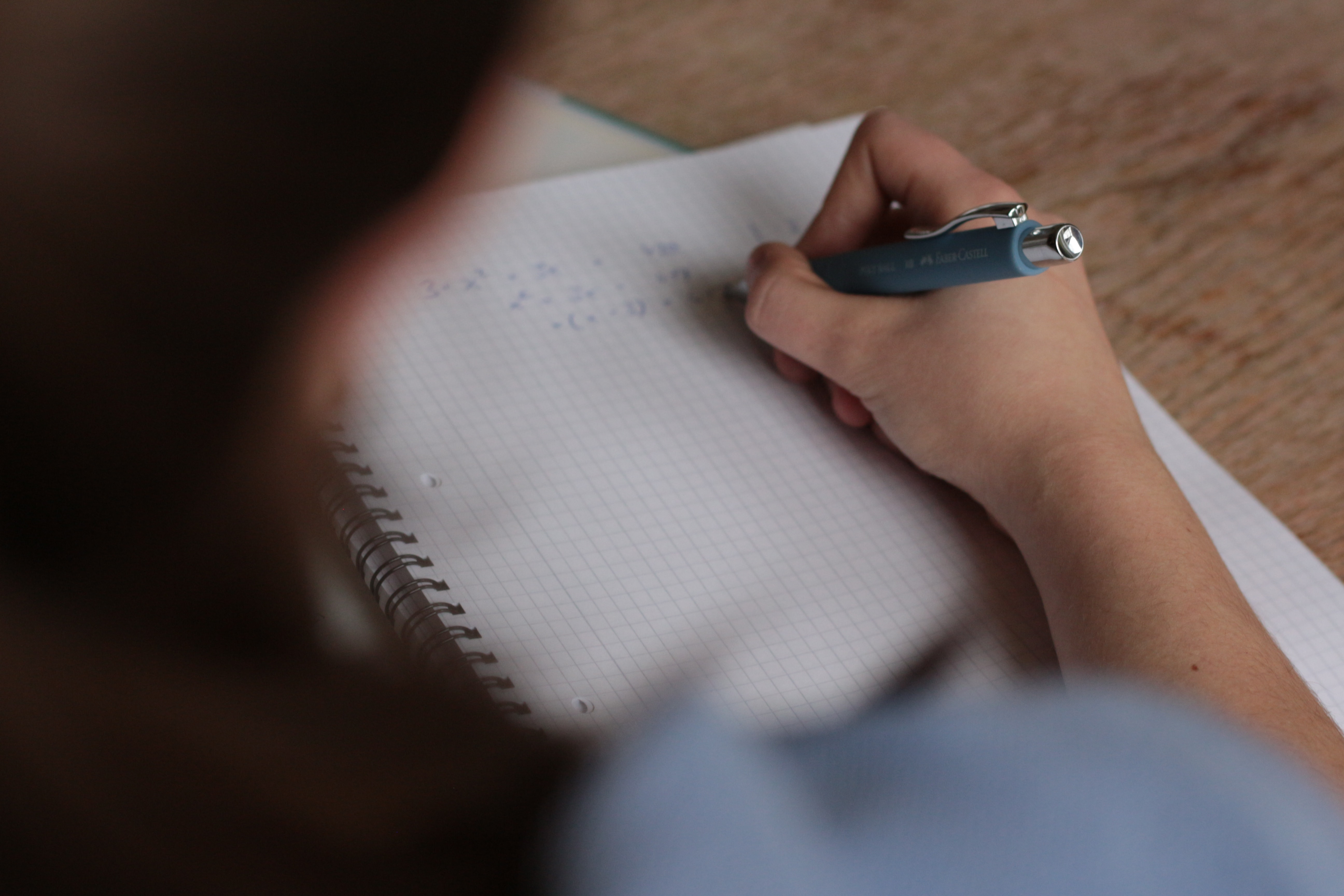# Solution to problems – class 9 – Set 1 Q 26

Last updated on June 17th, 2019 at 09:13 am

## Problem Statement

A race car accelerates uniformly from 25 m/s to 50 m/s in 5 seconds. Determine the acceleration of the car and the distance traveled.

## Solution

Acceleration a = (50-25)/5
a = 5 m/s2

To find out the distance travelled we will use the equation
V2 = U2 + 2 a S

where S=( V2 – U2 ) /2 a

S = (502 – 252)/(2 . 5) = 187.5 m

Question Set – Physics Problems for grade 9